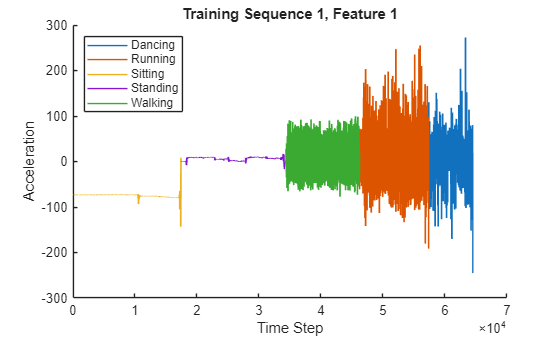# Sequence-to-Sequence Classification Using Deep Learning

This example shows how to classify each time step of sequence data using a long short-term memory (LSTM) network.

To train a deep neural network to classify each time step of sequence data, you can use a sequence-to-sequence LSTM network. A sequence-to-sequence LSTM network enables you to make different predictions for each individual time step of the sequence data.

This example uses sensor data obtained from a smartphone worn on the body. The example trains an LSTM network to recognize the activity of the wearer given time series data representing accelerometer readings in three different directions. The training data contains time series data for seven people. Each sequence has three features and varies in length. The data set contains six training observations and one test observation.

### Load Sequence Data

Load the human activity recognition data. The training data contains six sequences of sensor data obtained from a smartphone worn on the body. Each sequence has three features and varies in length. The three features correspond to the accelerometer readings in three different directions.

```load HumanActivityTrain XTrain```
```XTrain=6×1 cell array {3×64480 double} {3×53696 double} {3×56416 double} {3×50688 double} {3×51888 double} {3×54256 double} ```

Visualize one training sequence in a plot. Plot the first feature of the first training sequence and color the plot according to the corresponding activity.

```X = XTrain{1}(1,:); classes = categories(YTrain{1}); figure for j = 1:numel(classes) label = classes(j); idx = find(YTrain{1} == label); hold on plot(idx,X(idx)) end hold off xlabel("Time Step") ylabel("Acceleration") title("Training Sequence 1, Feature 1") legend(classes,'Location','northwest')```### Define LSTM Network Architecture

Define the LSTM network architecture. Specify the input to be sequences of size 3 (the number of features of the input data). Specify an LSTM layer with 200 hidden units, and output the full sequence. Finally, specify five classes by including a fully connected layer of size 5, followed by a softmax layer and a classification layer.

```numFeatures = 3; numHiddenUnits = 200; numClasses = 5; layers = [ ... sequenceInputLayer(numFeatures) lstmLayer(numHiddenUnits,'OutputMode','sequence') fullyConnectedLayer(numClasses) softmaxLayer classificationLayer];```

Specify the training options. Set the solver to `'adam'`. Train for 60 epochs. To prevent the gradients from exploding, set the gradient threshold to 2.

```options = trainingOptions('adam', ... 'MaxEpochs',60, ... 'GradientThreshold',2, ... 'Verbose',0, ... 'Plots','training-progress');```

Train the LSTM network with the specified training options using `trainNetwork`. Each mini-batch contains the whole training set, so the plot is updated once per epoch. The sequences are very long, so it might take some time to process each mini-batch and update the plot.

`net = trainNetwork(XTrain,YTrain,layers,options);`### Test LSTM Network

Load the test data and classify the activity at each time step.

Load the human activity test data. `XTest` contains a single sequence of dimension 3. `YTest` is contains sequence of categorical labels corresponding to the activity at each time step.

```load HumanActivityTest figure plot(XTest{1}') xlabel("Time Step") legend("Feature " + (1:numFeatures)) title("Test Data")```Classify the test data using `classify`.

`YPred = classify(net,XTest{1});`

Alternatively, you can make predictions one time step at a time by using `classifyAndUpdateState`. This is useful when you have the values of the time steps arriving in a stream. Usually, it is faster to make predictions on full sequences when compared to making predictions one time step at a time. For an example showing how to forecast future time steps by updating the network between single time step predictions, see Time Series Forecasting Using Deep Learning.

Calculate the accuracy of the predictions.

`acc = sum(YPred == YTest{1})./numel(YTest{1})`
```acc = 0.9998 ```

Compare the predictions with the test data by using a plot.

```figure plot(YPred,'.-') hold on plot(YTest{1}) hold off xlabel("Time Step") ylabel("Activity") title("Predicted Activities") legend(["Predicted" "Test Data"])```